# Predicting Churn with KNN

Although there are many examples, churn prediction is one of the classical applications of Data Science that works. Churn prediction gives businessmen and bussinesswomen the power to catch those consumers who are likely to leave the company. They can in turn take appropriate measure to keep their business. In this project, we consider a dataset of 7,043 observations. The goal here is to predict, with a fair amount of accuracy, which observations are likely to churn.

## Exploratory Data Analysis

``## Loading required package: ggplot2``
``## Loading required package: magrittr``

Note that the `echo = FALSE` parameter was added to the code chunk to prevent printing of the R code that generated the plot.

``DT::datatable(churn[,c(1:2,16:21)])``

## Including Plots

You can also embed plots, for example:

``````my.data <- cbind(churn,data.frame(num = 1) )
my.data\$SeniorCitizen <- factor(my.data\$SeniorCitizen)
my.data2 <- aggregate(num~. , data = my.data[, c(7:8, 12, 16,21, 22)], sum)

SankeyDiagram(my.data2[, - dim(my.data2)],
weights = my.data2\$num) ``````
``````temp <- churn[churn\$Churn == "Yes", ]
var <- temp\$Contract  # the categorical data

nrows <- 10
df <- expand.grid(y = 1:nrows, x = 1:nrows)
categ_table <- round(table(var) * ((nrows*nrows)/(length(var))))
categ_table <- categ_table - 1 #Adjust

df\$category <- factor(rep(names(categ_table), categ_table))
# NOTE: if sum(categ_table) is not 100 (i.e. nrows^2), it will need adjustment to make the sum to 100.

## Plot
ggplot(df, aes(x = x, y = y, fill = category)) +
geom_tile(color = "black", size = 0.5) +
scale_x_continuous(expand = c(0, 0)) +
scale_y_continuous(expand = c(0, 0), trans = 'reverse') +
scale_fill_brewer(palette = "Set3") +
labs(title="Customer churn % (+)", subtitle="Contract Type",
caption="Telecom") +
theme(
plot.title = element_text(size = rel(1.2)),
axis.text = element_blank(),
axis.title = element_blank(),
axis.ticks = element_blank(),
legend.title = element_blank(),
legend.position = "right")``````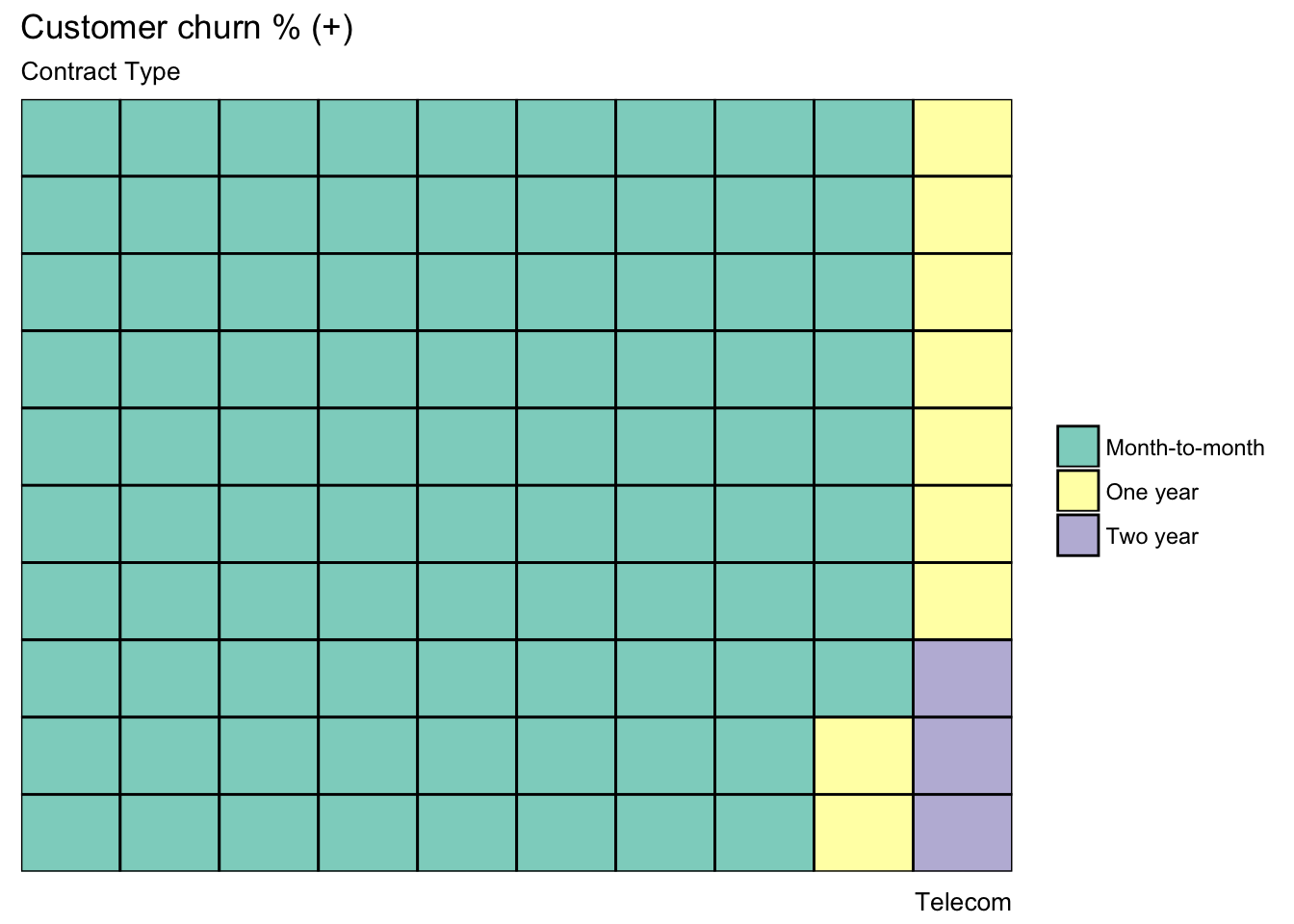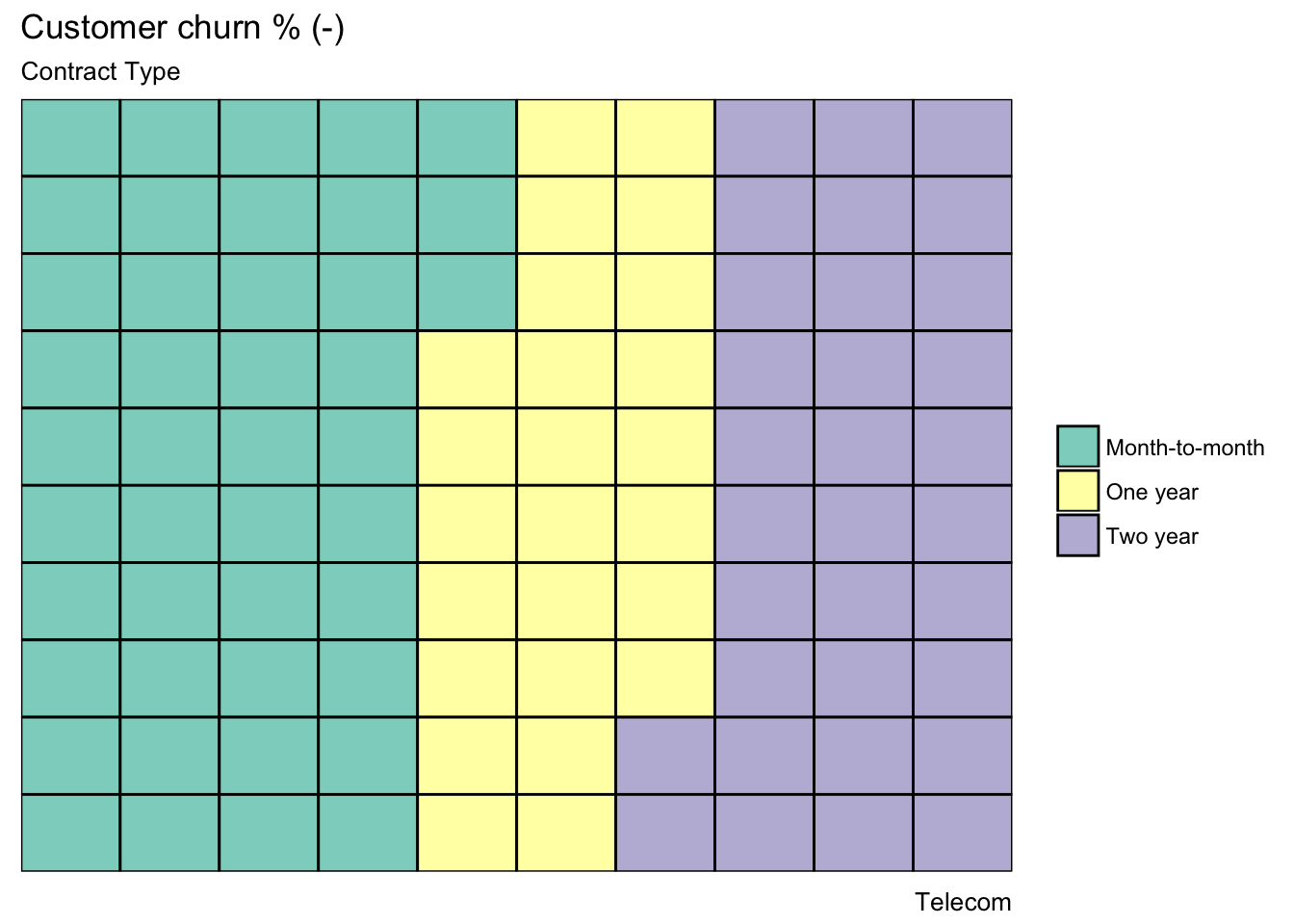### Exploring

``````plot.barplot(x = churn,
xname = "Churn",
fillname = "Contract")``````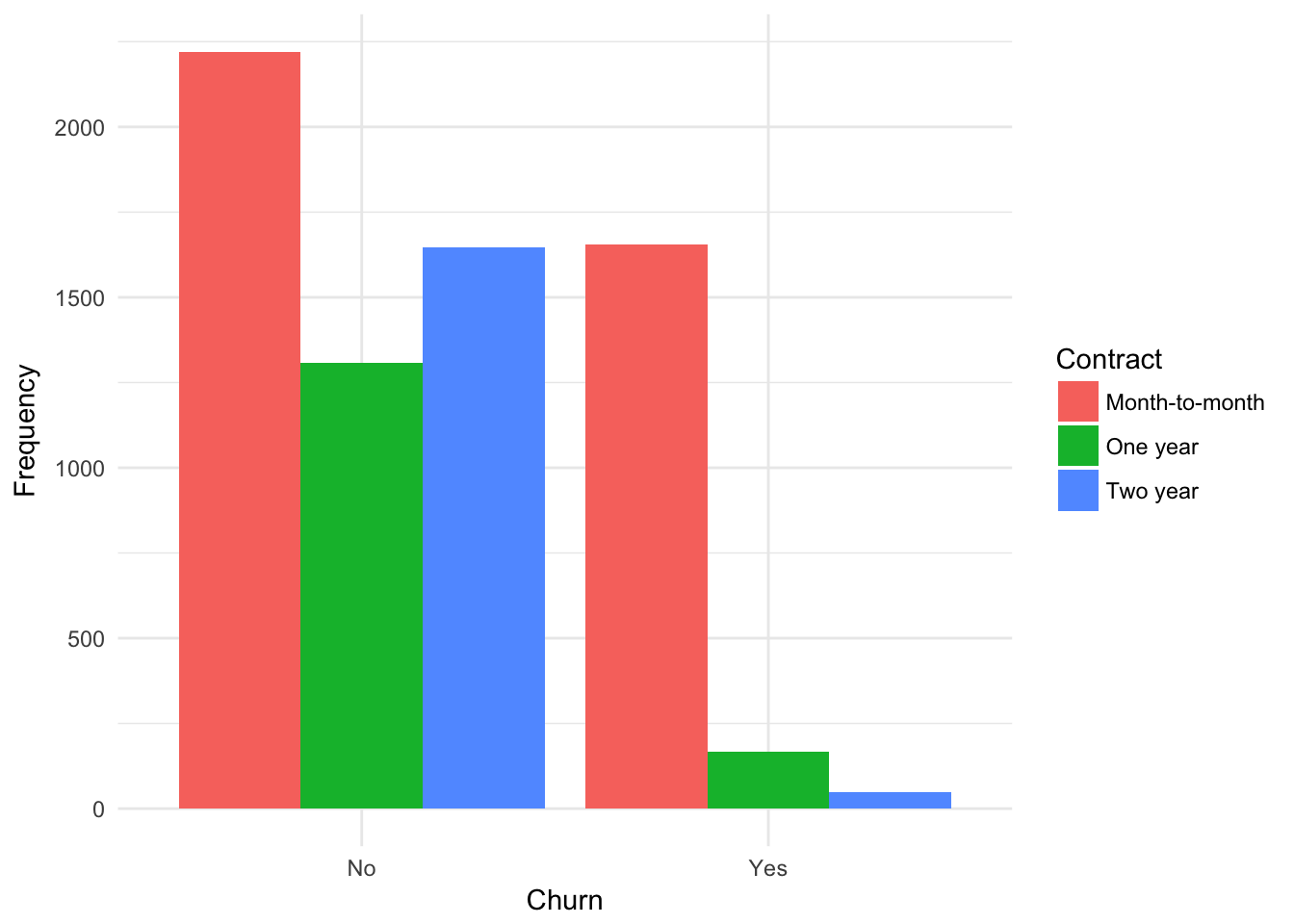``````##
##       Month-to-month One year Two year
##   No            2220     1307     1647
##   Yes           1655      166       48``````
``````plot.barplot(churn,
xname = "Churn" ,
fillname = "Dependents")``````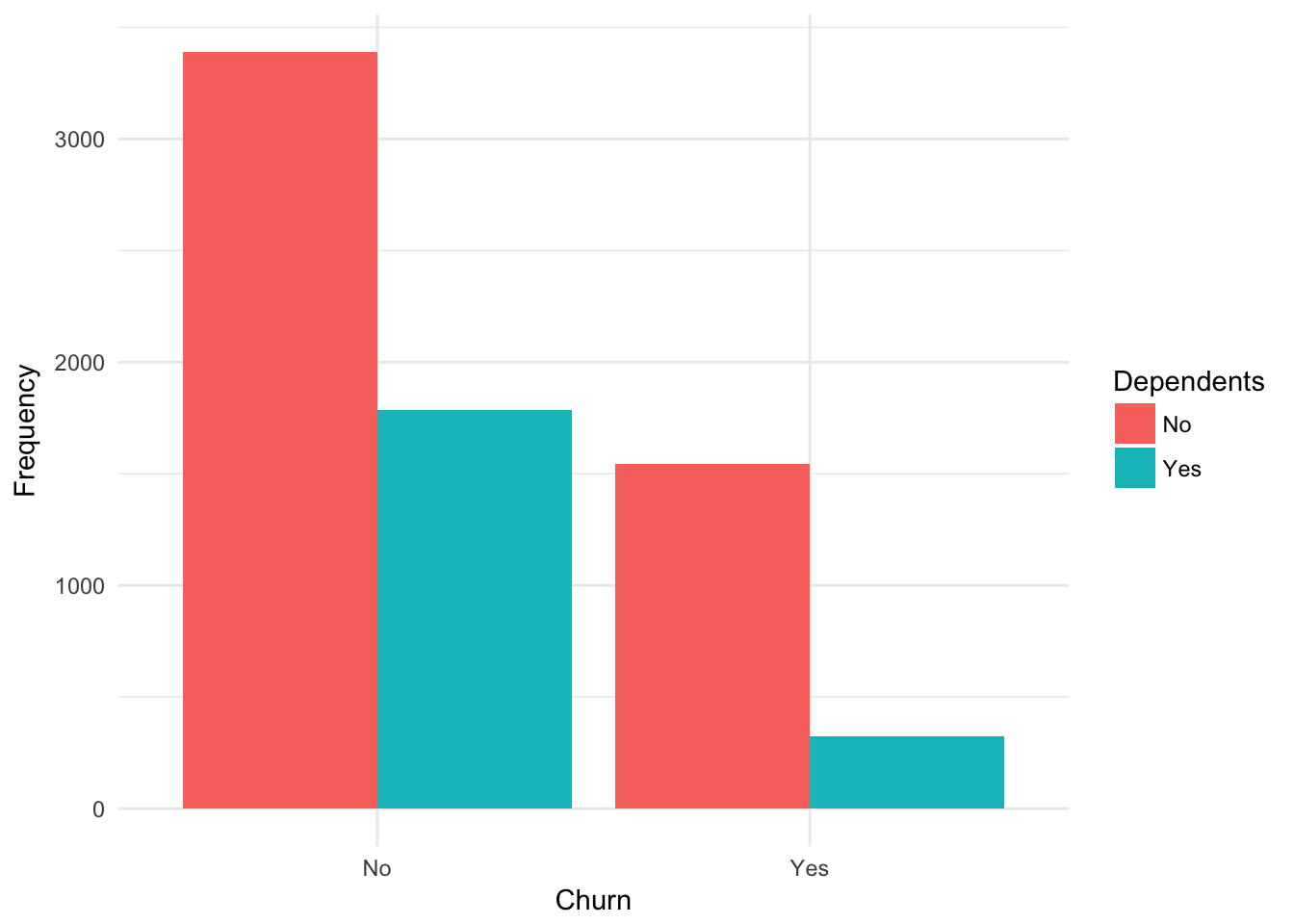``````##
##         No  Yes
##   No  3390 1784
##   Yes 1543  326``````
``````plot.barplot(churn,
xname = "Churn",
fillname = "StreamingMovies")``````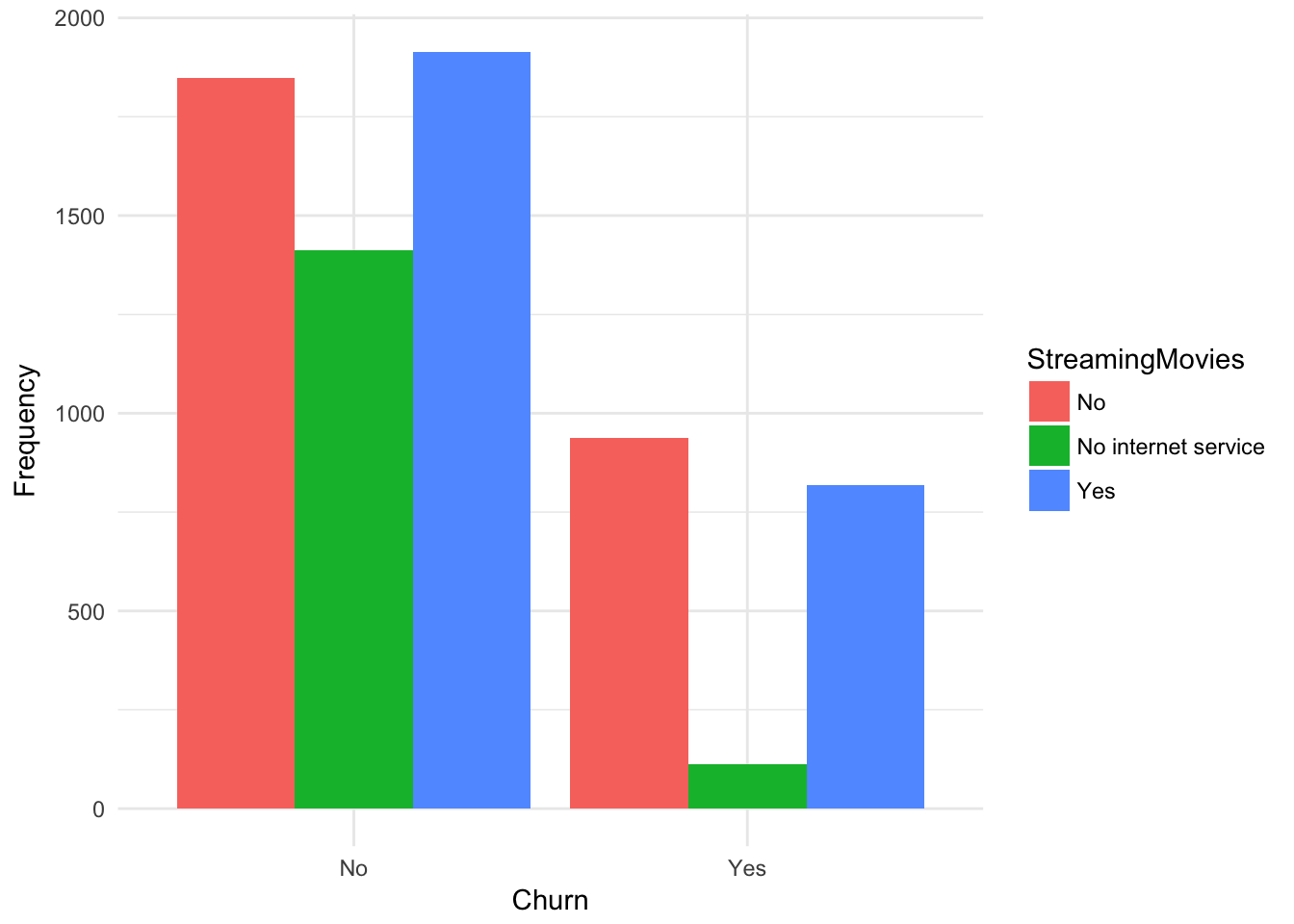``````##
##         No No internet service  Yes
##   No  1847                1413 1914
##   Yes  938                 113  818``````
``````plot.barplot(churn,
xname = "Churn",
fillname = "PaymentMethod") ``````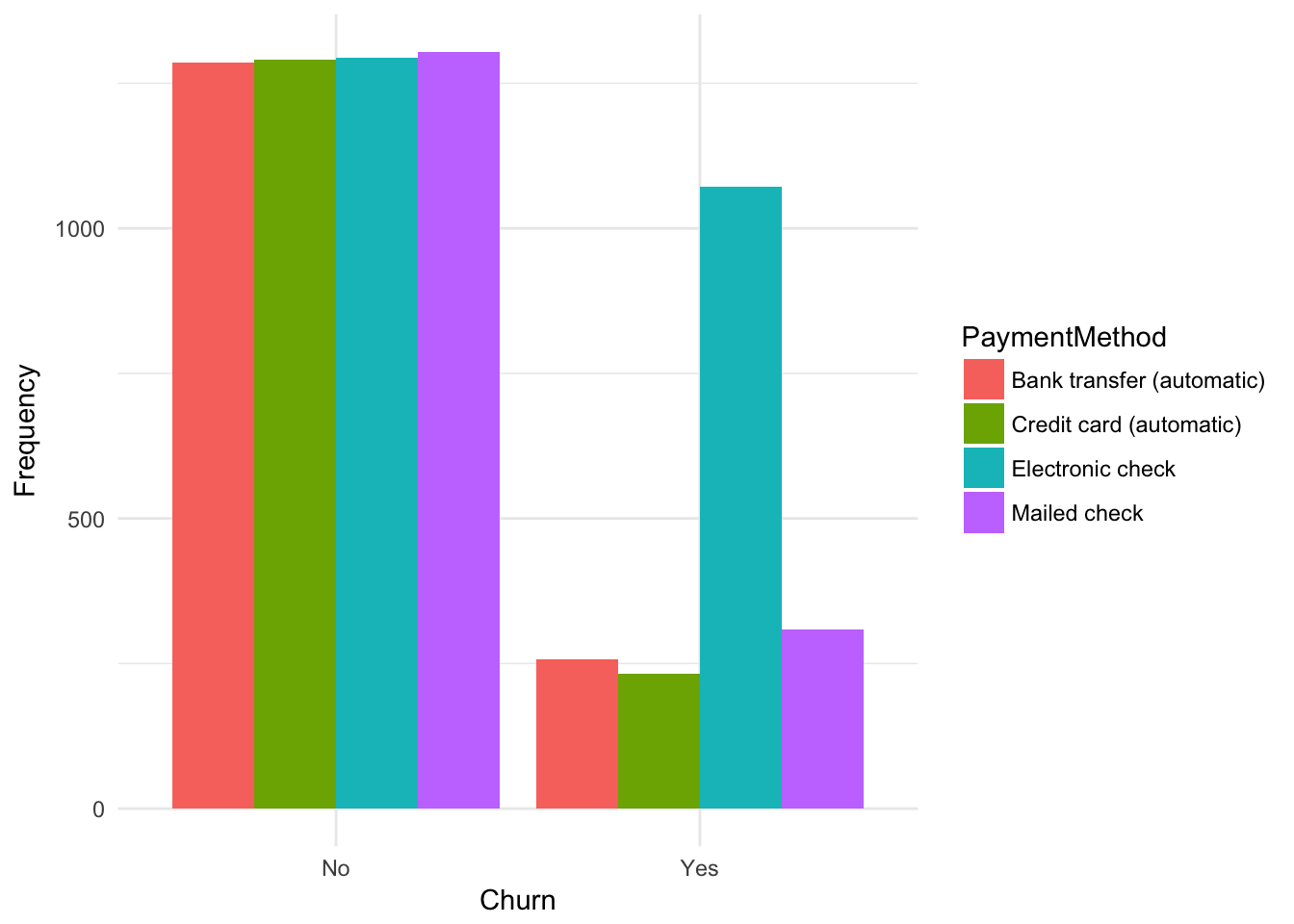``````##
##       Bank transfer (automatic) Credit card (automatic) Electronic check
##   No                       1286                    1290             1294
##   Yes                       258                     232             1071
##
##       Mailed check
##   No          1304
##   Yes          308``````
``````ggplot(churn, aes(x = Churn, y = tenure, fill= Contract)) +
geom_boxplot() + theme_minimal()``````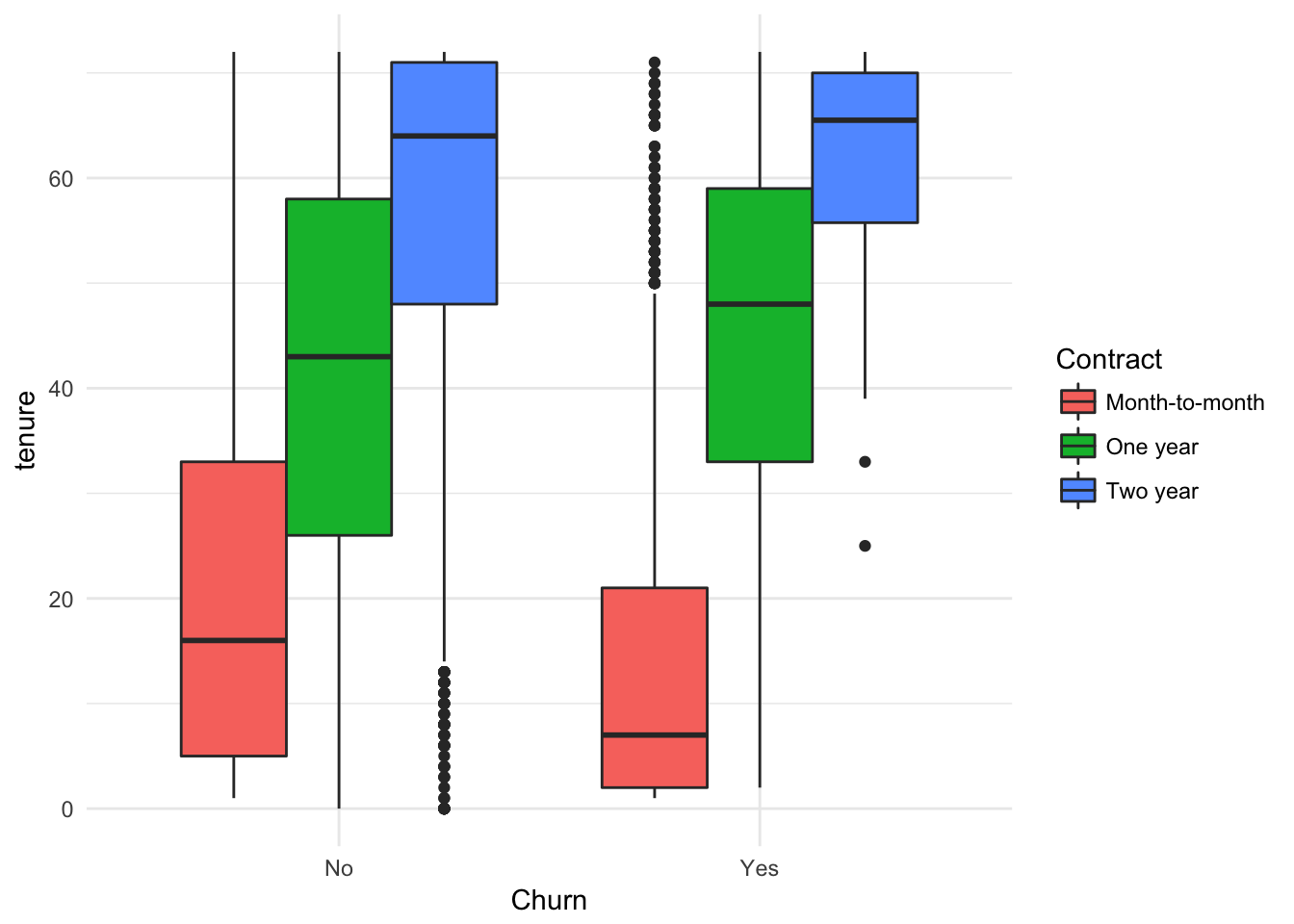``````ggplot(churn, aes(x = Churn, y = tenure)) +
geom_boxplot() + theme_minimal()``````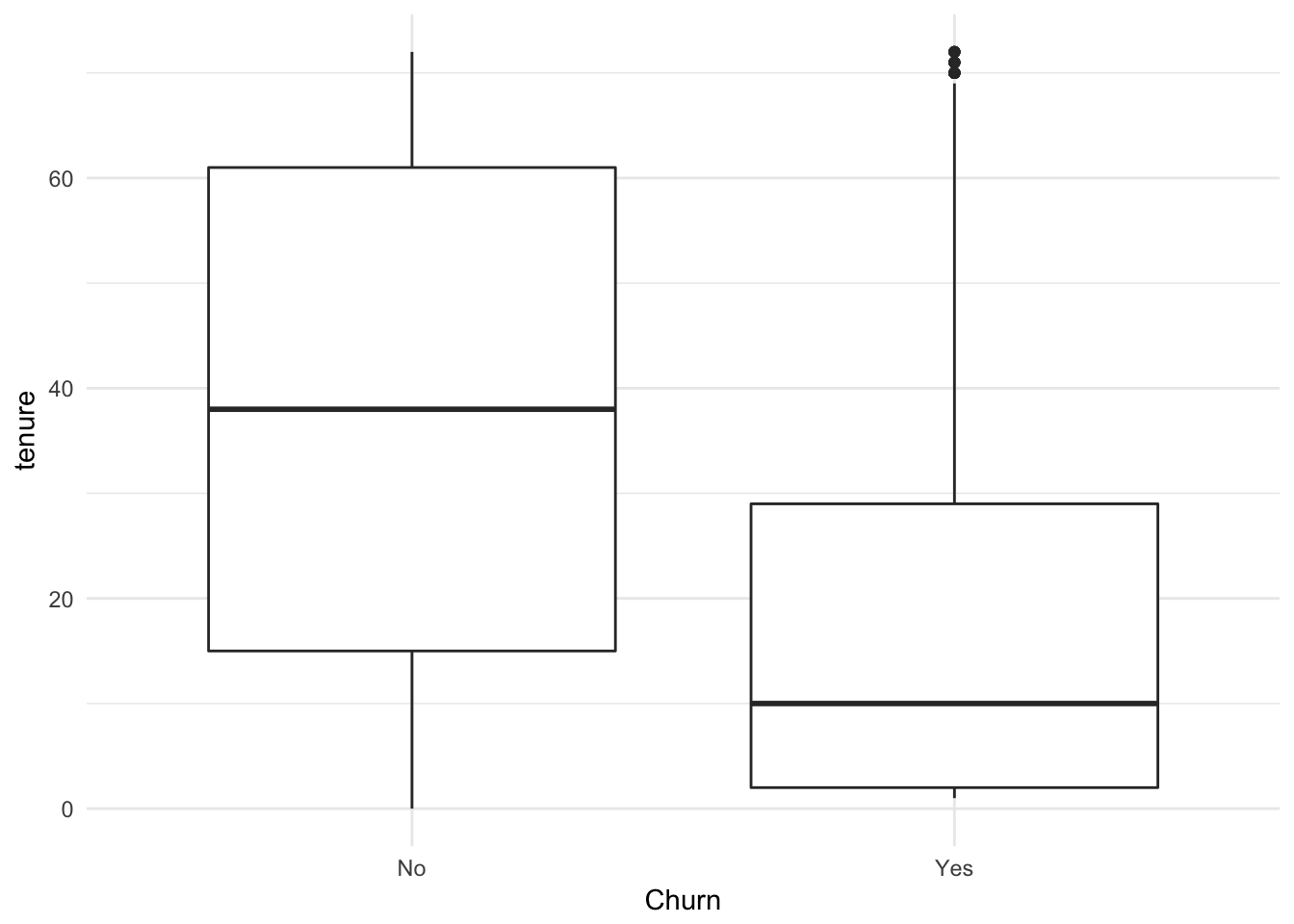``````ggplot(churn, aes(x = Churn, y = tenure, fill= TechSupport)) +
geom_boxplot() + facet_wrap(~SeniorCitizen)+ theme_minimal()``````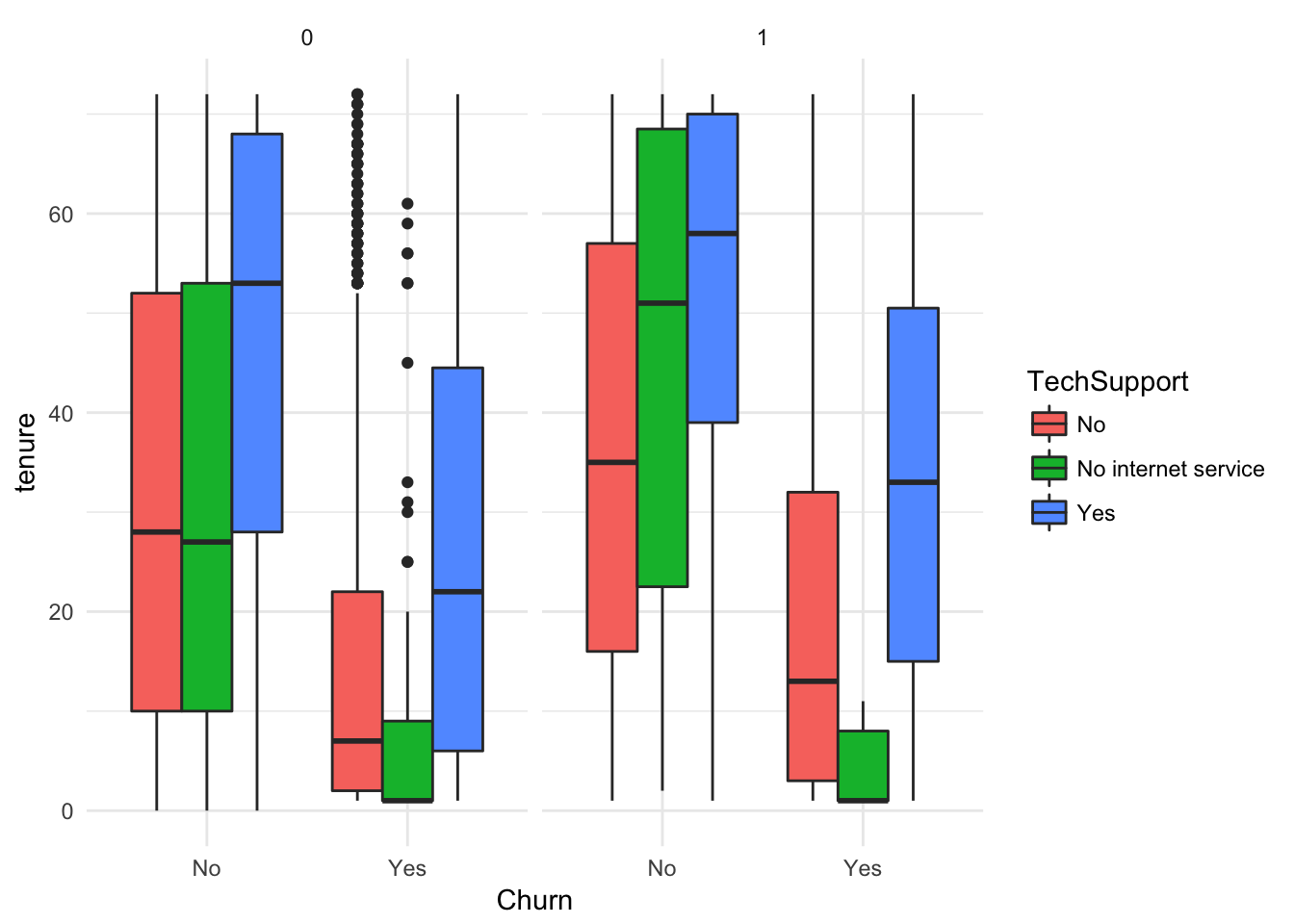``````ggplot(churn, aes(x = Churn, y = MonthlyCharges, fill= Contract)) +
geom_boxplot() + theme_minimal()``````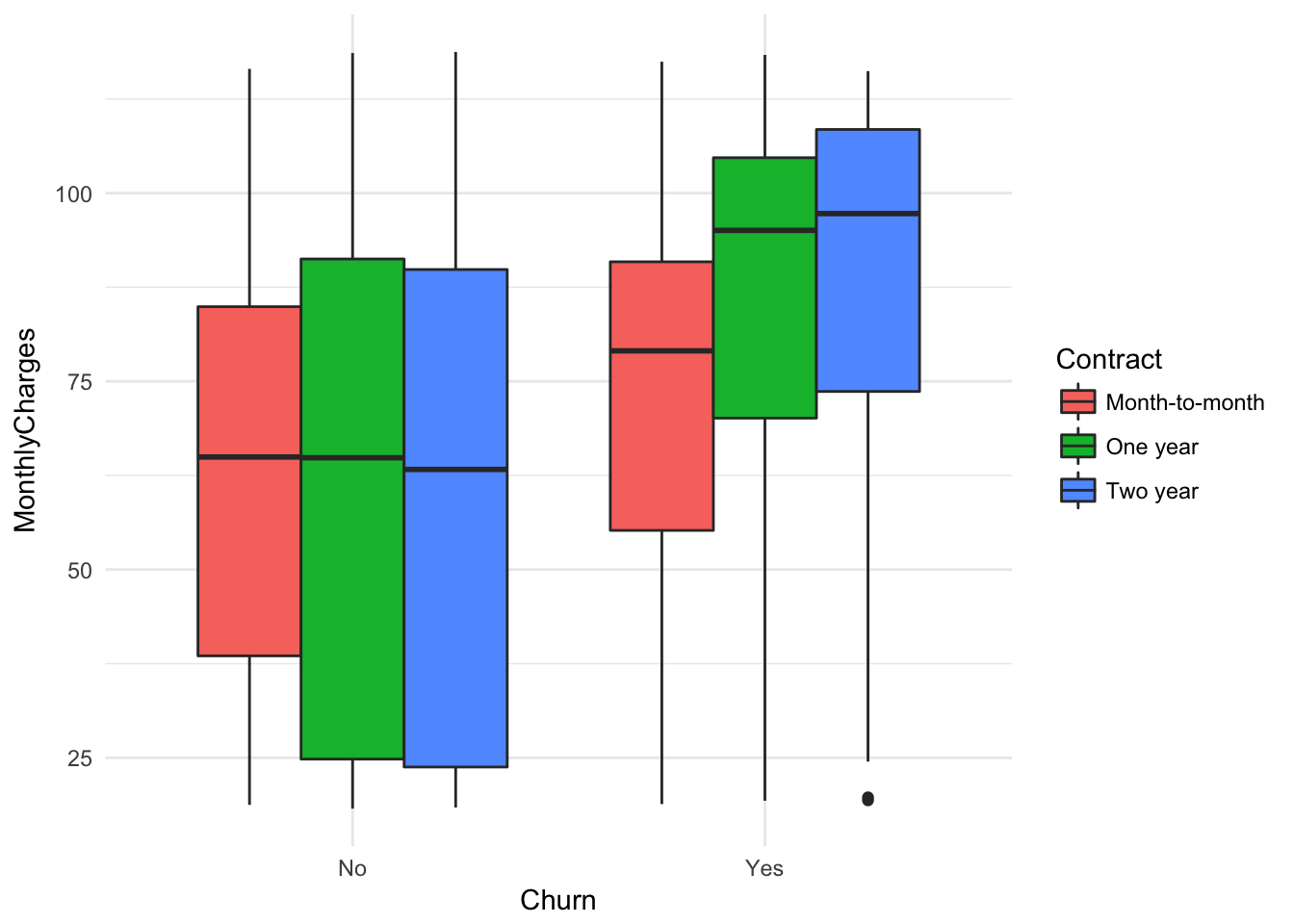``ggplot(churn, aes(x = tenure, y = TotalCharges, color = Contract)) + geom_point( ) + geom_smooth()+ theme_minimal()``
``## `geom_smooth()` using method = 'gam'``
``## Warning: Removed 11 rows containing non-finite values (stat_smooth).``
``## Warning: Removed 11 rows containing missing values (geom_point).``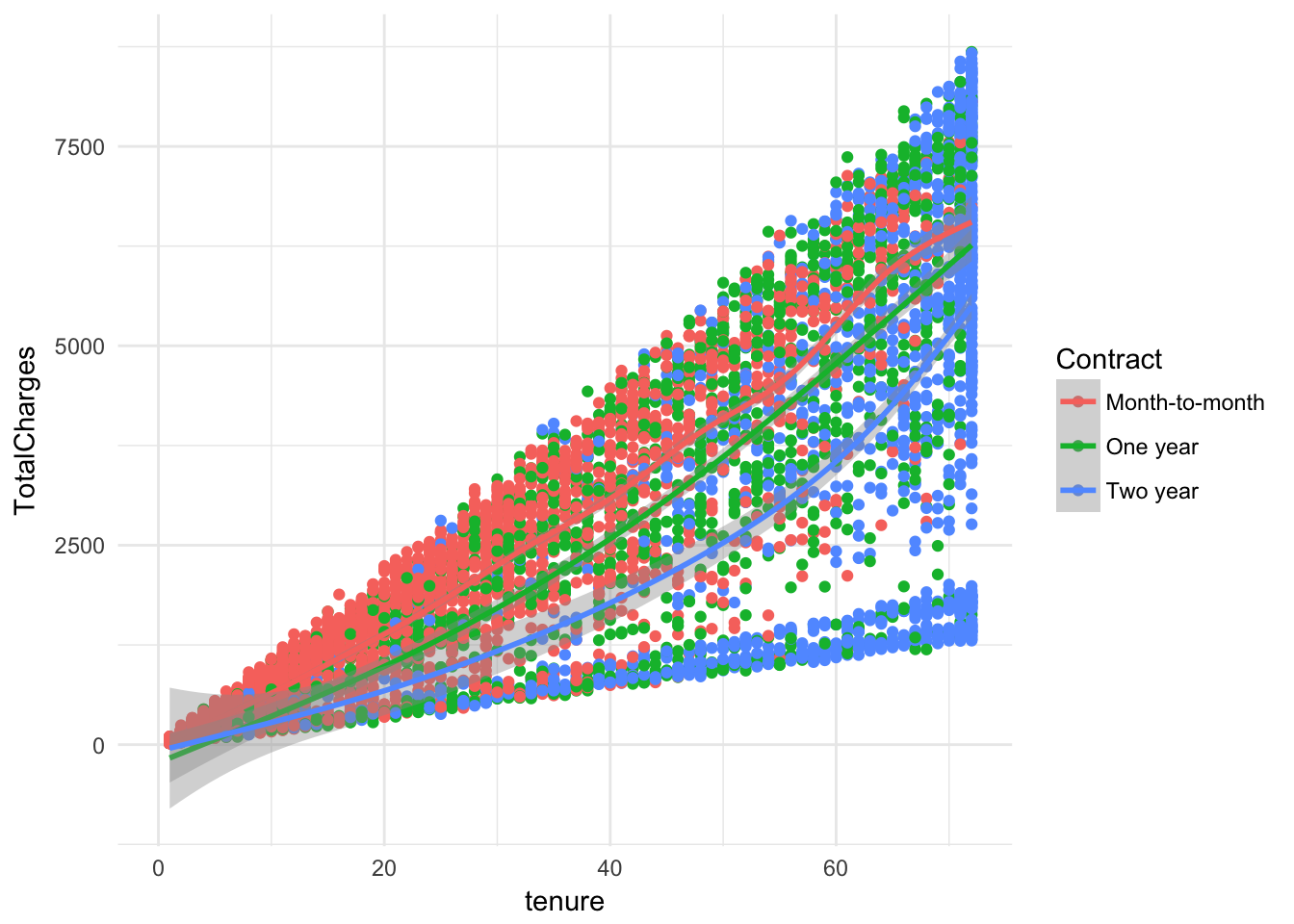``ggplot(churn, aes(x = MonthlyCharges, y = TotalCharges, color = Contract)) + geom_point( ) + geom_smooth()+ theme_minimal()``
``## `geom_smooth()` using method = 'gam'``
``````## Warning: Removed 11 rows containing non-finite values (stat_smooth).

## Warning: Removed 11 rows containing missing values (geom_point).``````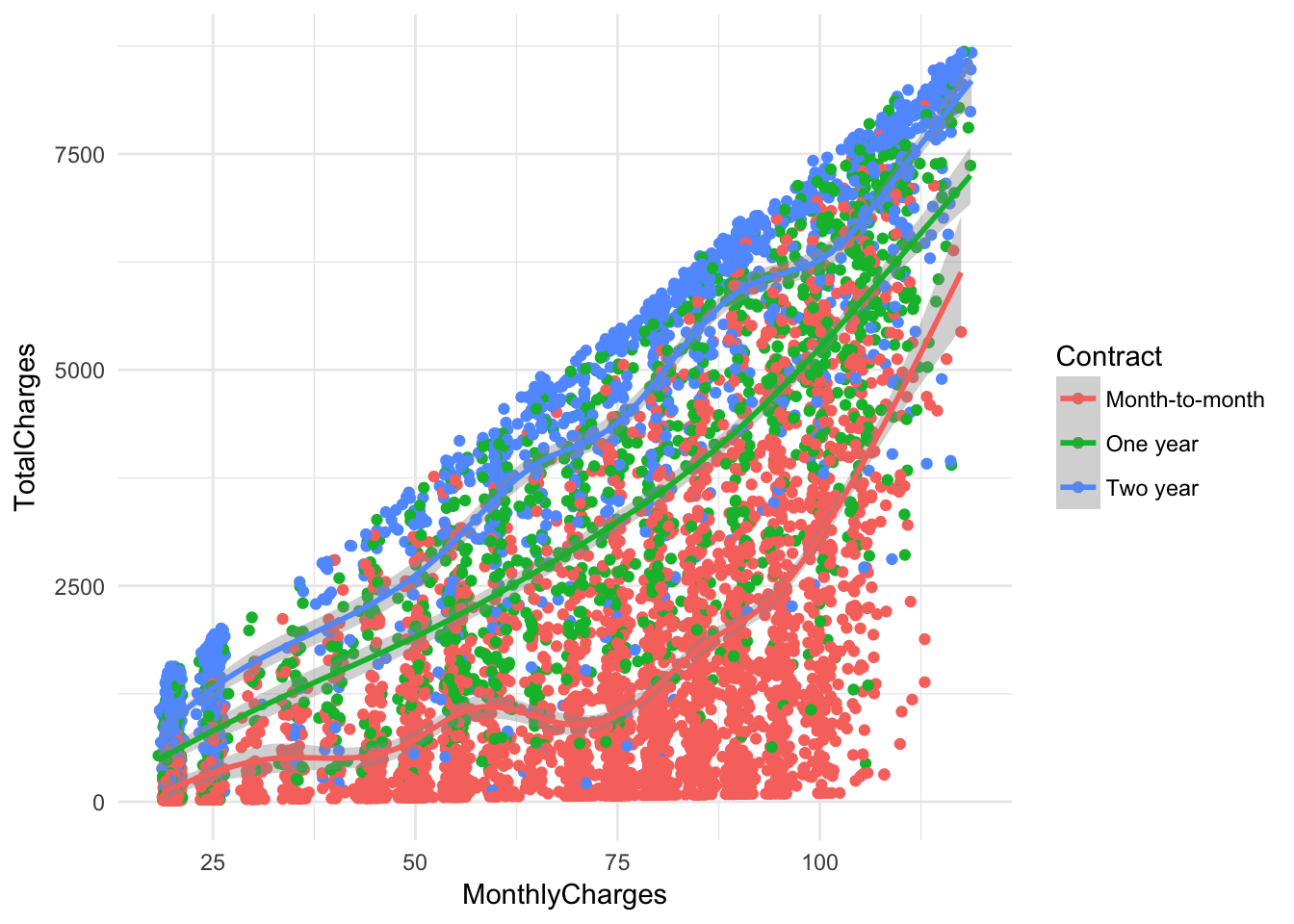``````ggplot(churn, aes(x = tenure, y = MonthlyCharges, color = Churn)) +
geom_point( )+ geom_smooth()+ facet_wrap(~factor(InternetService))+ theme_minimal()``````
``## `geom_smooth()` using method = 'gam'``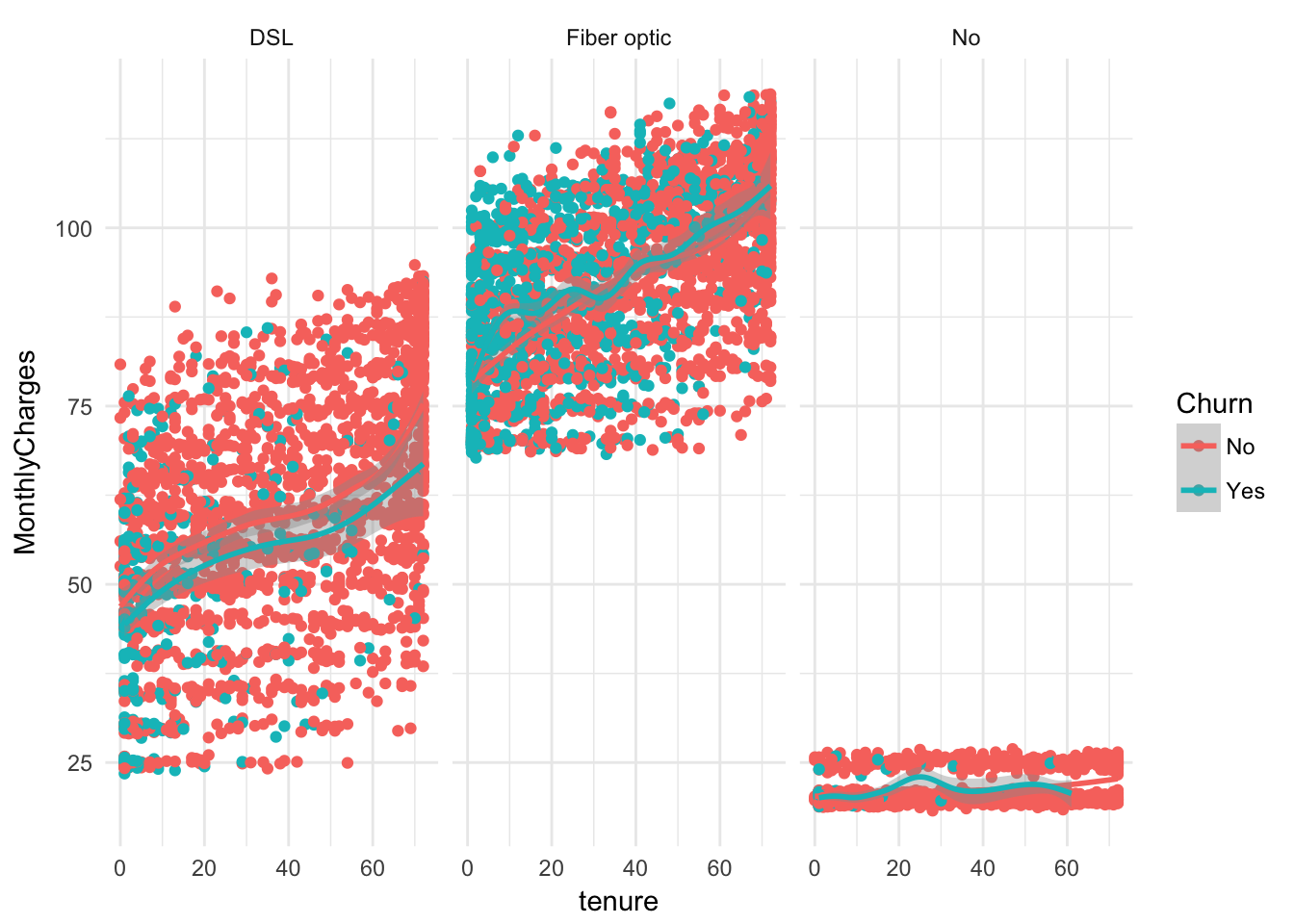``````ggplot(churn, aes(x = Churn, y = tenure, fill = InternetService)) +
geom_boxplot() + facet_wrap(~TechSupport, nrow = 1)+ theme_minimal()``````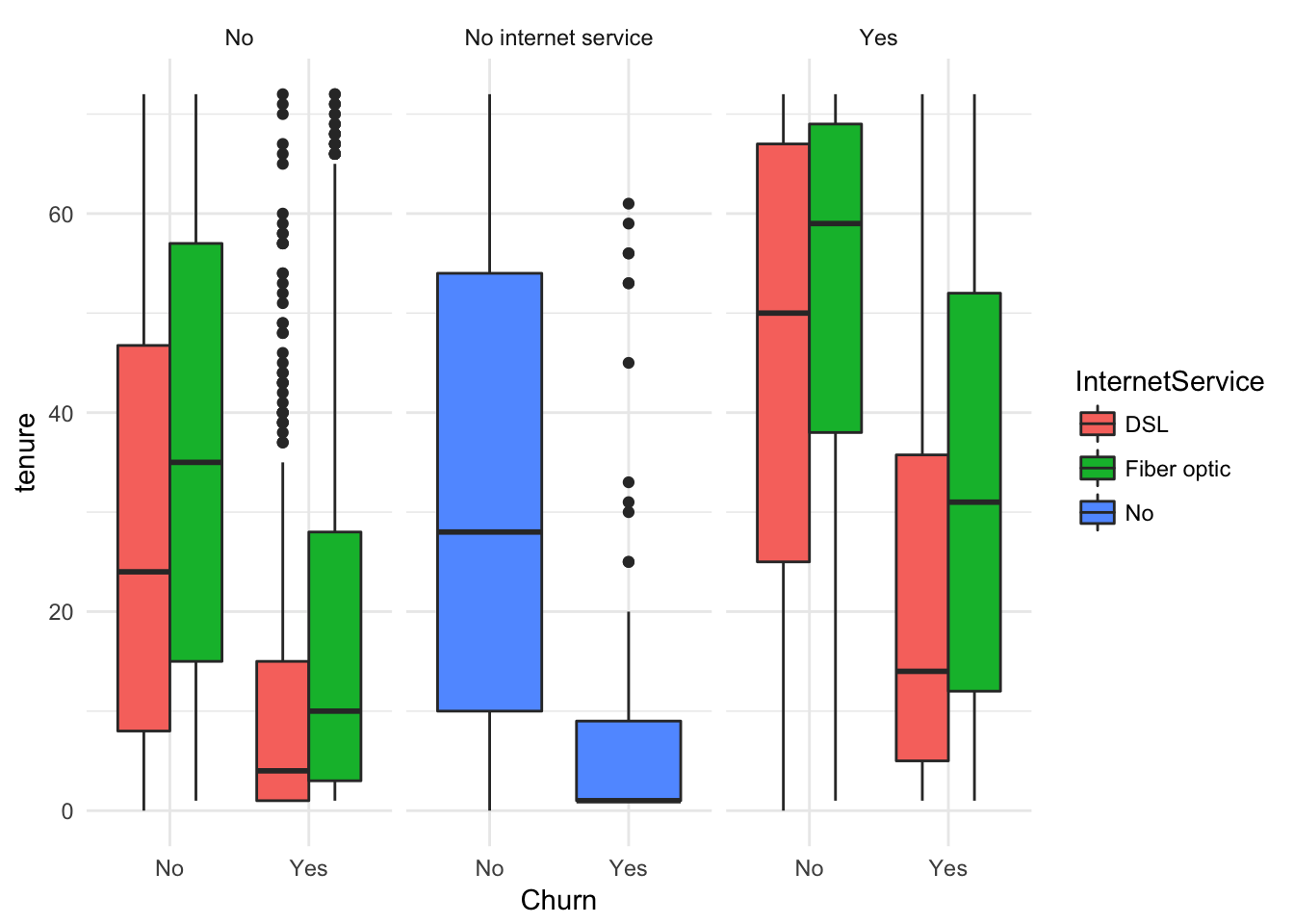``````ggplot(churn, aes(x = MonthlyCharges, fill = Churn)) +
geom_histogram() + theme_minimal()#Monthly Charges by Churn``````
``## `stat_bin()` using `bins = 30`. Pick better value with `binwidth`.``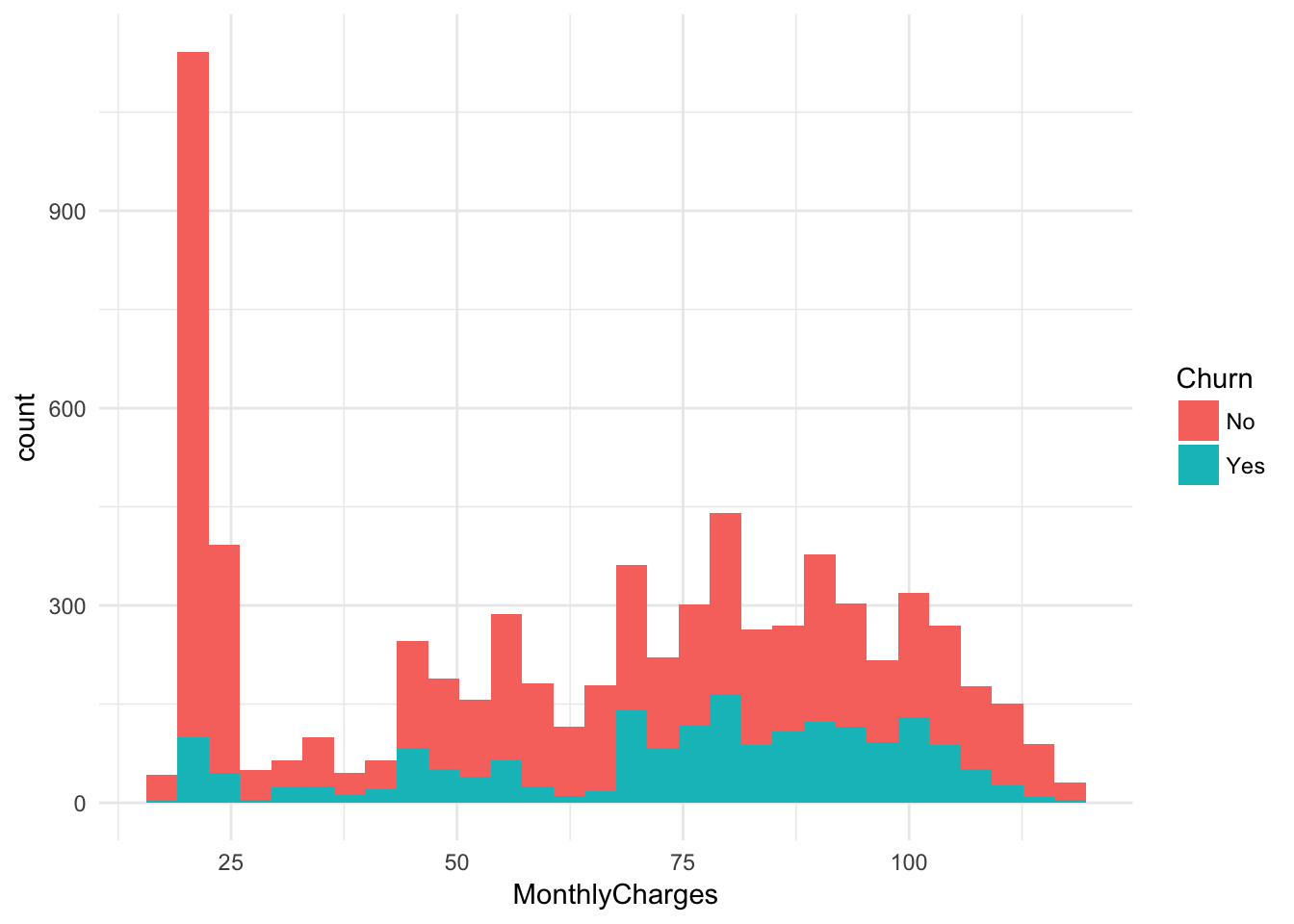``````ggplot(churn, aes(x = MonthlyCharges, fill = TechSupport)) +
geom_histogram() + theme_minimal()``````
``## `stat_bin()` using `bins = 30`. Pick better value with `binwidth`.``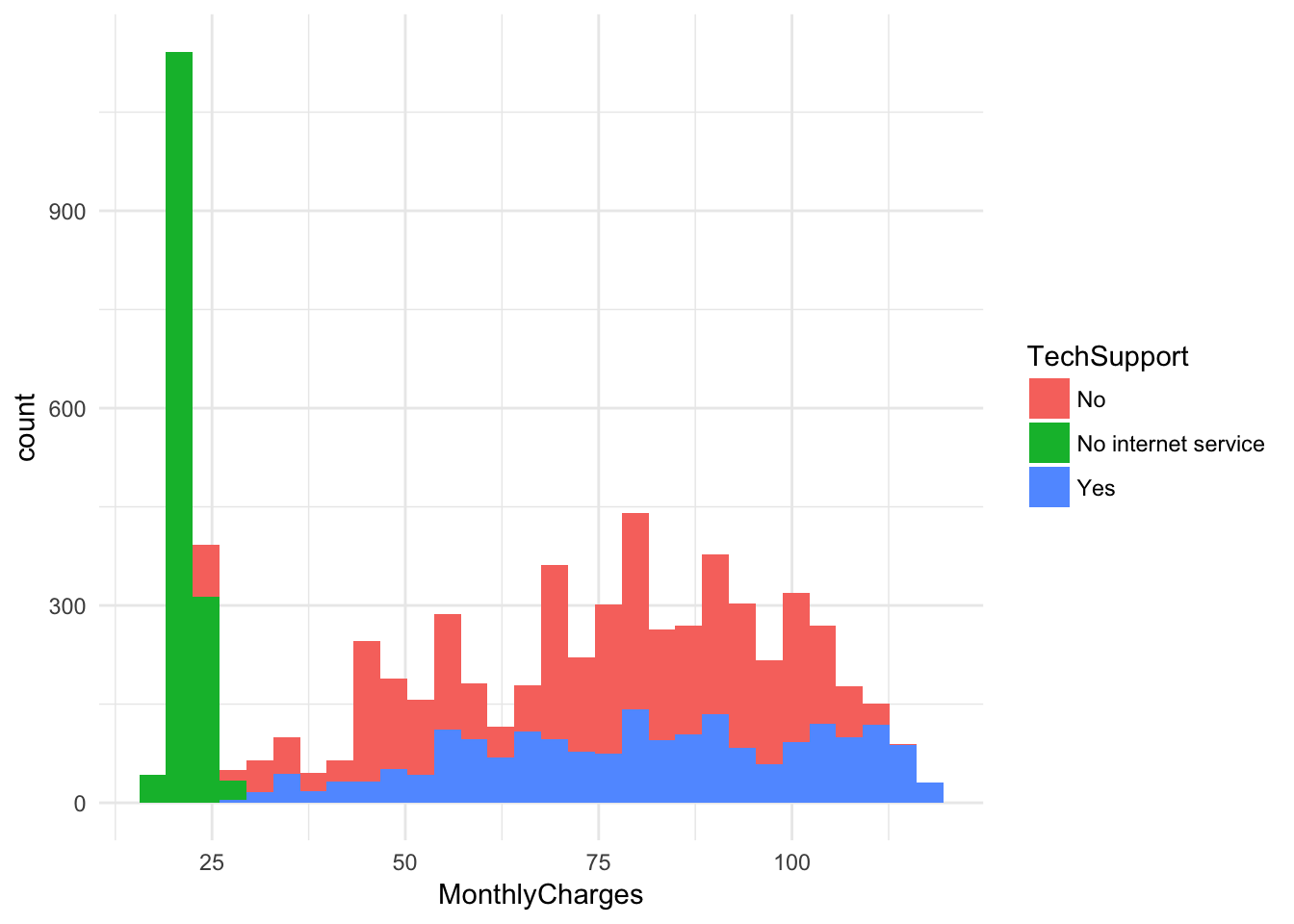``````ggplot(churn, aes(x = MonthlyCharges, fill = InternetService)) +
geom_histogram() + theme_minimal()``````
``## `stat_bin()` using `bins = 30`. Pick better value with `binwidth`.``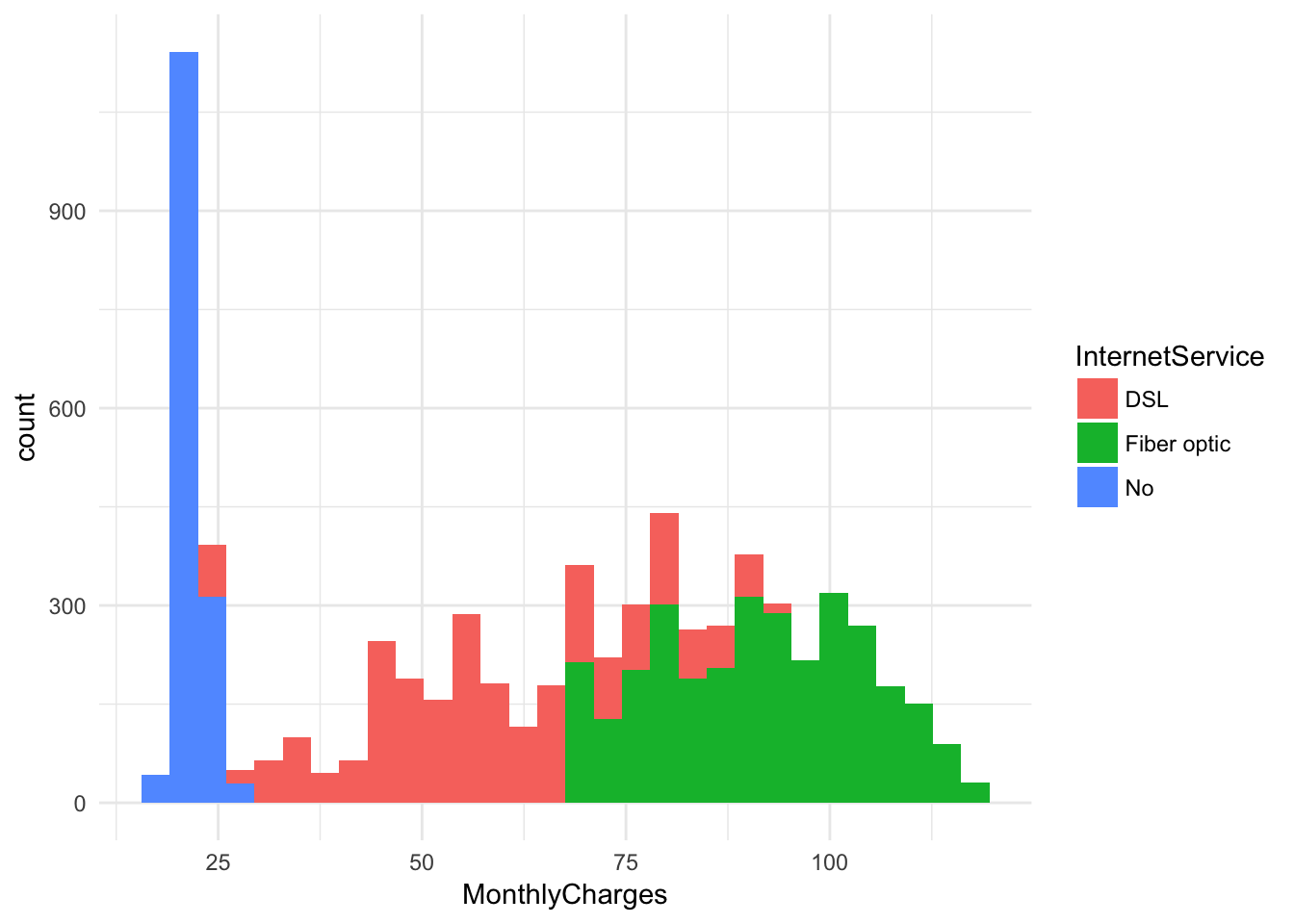``````ggplot(churn, aes(x = TotalCharges, fill = Contract)) +
geom_histogram() + facet_wrap(~InternetService) + theme_minimal()``````
``## `stat_bin()` using `bins = 30`. Pick better value with `binwidth`.``
``## Warning: Removed 11 rows containing non-finite values (stat_bin).``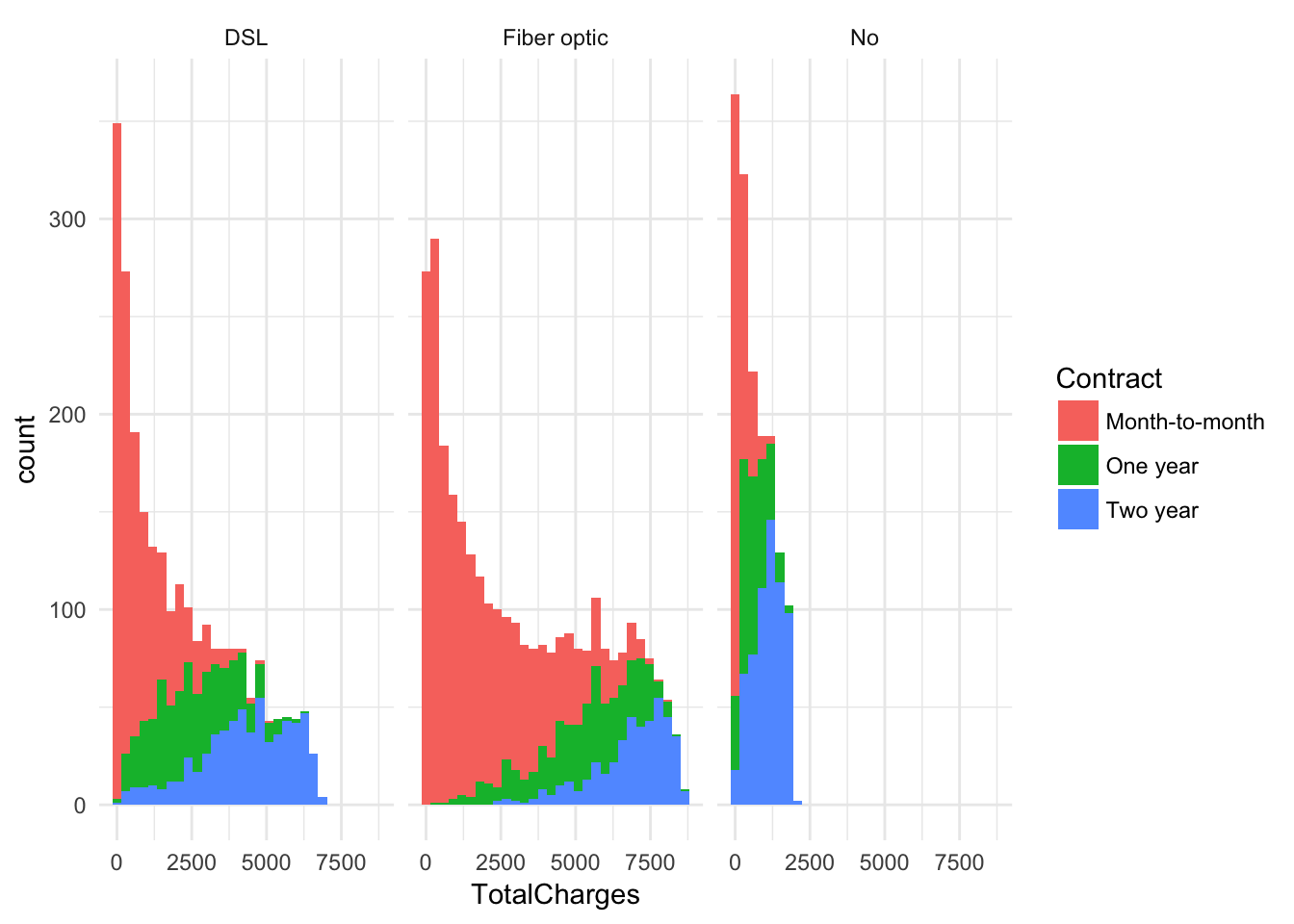`````` ggplot(churn, aes(x = tenure, y = MonthlyCharges, color = InternetService)) +
geom_point( )+ geom_smooth()+ theme_minimal()``````
``## `geom_smooth()` using method = 'gam'``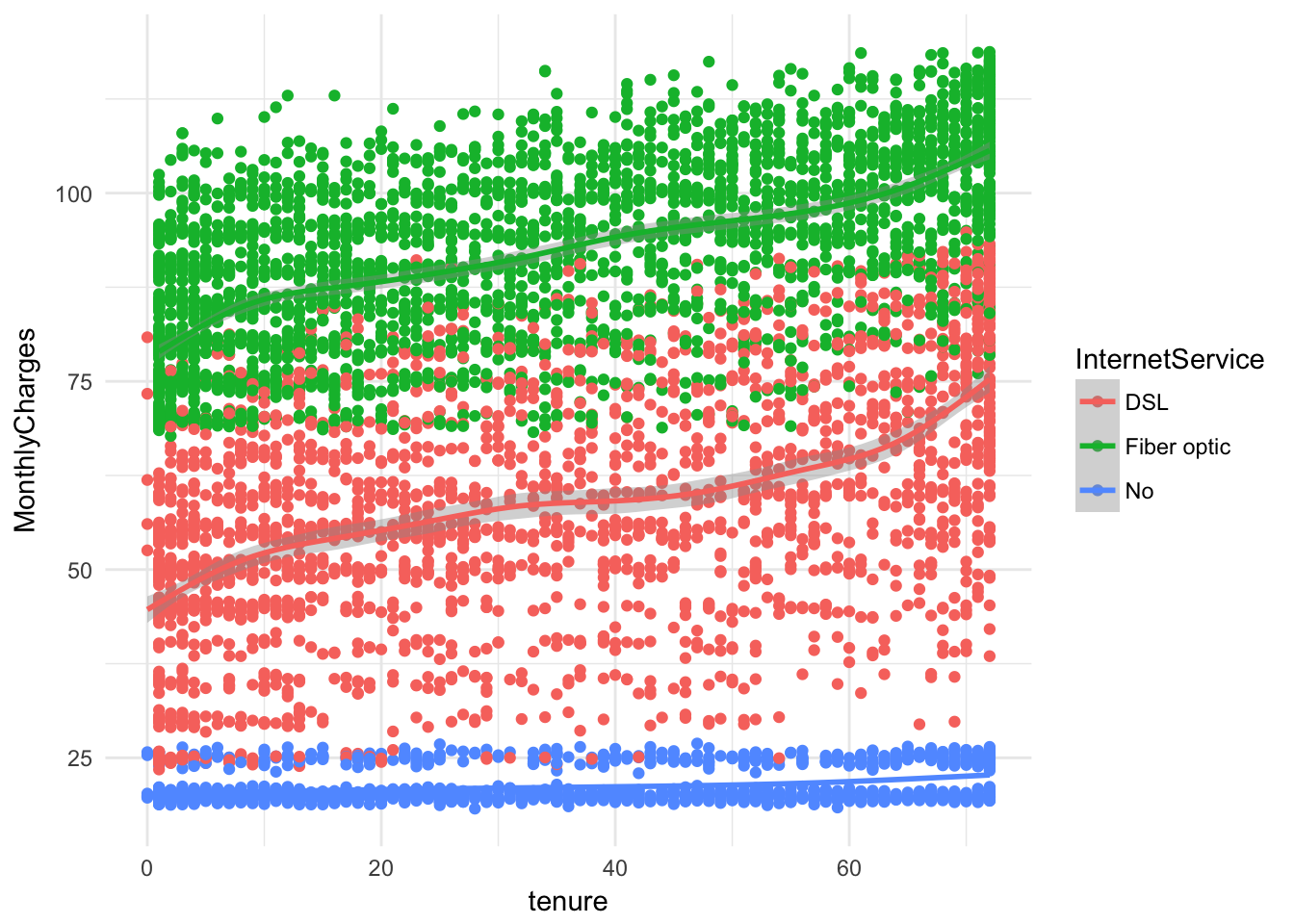## Model Building

For this mini-project, we will use logistic regression, decision trees, and k-nearest neighbors.

``````set.seed(100)
indx <- sample(1:nrow(churn), .8*nrow(churn), replace = F)``````

### Logistic Regression

Since I am only using logistic regression to explore relationships, and I’m not necessarily too concerned with the accuracy of this particular model, I will hold off on splitting the data 80-20 until the subsequent models.

``````churn\$Churn <- factor(churn\$Churn)
churn\$SeniorCitizen <- factor(churn\$SeniorCitizen )
fit <- glm(Churn ~ SeniorCitizen + MonthlyCharges + tenure + Contract +
PaymentMethod +TechSupport, data = churn, family = "binomial")
summary(fit)``````
``````##
## Call:
## glm(formula = Churn ~ SeniorCitizen + MonthlyCharges + tenure +
##     Contract + PaymentMethod + TechSupport, family = "binomial",
##     data = churn)
##
## Deviance Residuals:
##     Min       1Q   Median       3Q      Max
## -1.8322  -0.6735  -0.3045   0.7601   3.1709
##
## Coefficients:
##                                       Estimate Std. Error z value Pr(>|z|)
## (Intercept)                          -1.330859   0.149909  -8.878  < 2e-16
## SeniorCitizen1                        0.349465   0.081552   4.285 1.83e-05
## MonthlyCharges                        0.022466   0.001796  12.508  < 2e-16
## tenure                               -0.034080   0.002107 -16.174  < 2e-16
## ContractOne year                     -0.812642   0.103350  -7.863 3.75e-15
## ContractTwo year                     -1.560966   0.171277  -9.114  < 2e-16
## PaymentMethodCredit card (automatic) -0.060629   0.112223  -0.540 0.589023
## PaymentMethodElectronic check         0.424624   0.092594   4.586 4.52e-06
## PaymentMethodMailed check            -0.068929   0.111202  -0.620 0.535353
## TechSupportNo internet service       -0.484025   0.142733  -3.391 0.000696
## TechSupportYes                       -0.536799   0.082908  -6.475 9.51e-11
##
## (Intercept)                          ***
## SeniorCitizen1                       ***
## MonthlyCharges                       ***
## tenure                               ***
## ContractOne year                     ***
## ContractTwo year                     ***
## PaymentMethodCredit card (automatic)
## PaymentMethodElectronic check        ***
## PaymentMethodMailed check
## TechSupportNo internet service       ***
## TechSupportYes                       ***
## ---
## Signif. codes:  0 '***' 0.001 '**' 0.01 '*' 0.05 '.' 0.1 ' ' 1
##
## (Dispersion parameter for binomial family taken to be 1)
##
##     Null deviance: 8150.1  on 7042  degrees of freedom
## Residual deviance: 6000.7  on 7032  degrees of freedom
## AIC: 6022.7
##
## Number of Fisher Scoring iterations: 6``````
``exp(coef(fit))``
``````##                          (Intercept)                       SeniorCitizen1
##                            0.2642503                            1.4183090
##                       MonthlyCharges                               tenure
##                            1.0227206                            0.9664939
##                     ContractOne year                     ContractTwo year
##                            0.4436841                            0.2099333
## PaymentMethodCredit card (automatic)        PaymentMethodElectronic check
##                            0.9411728                            1.5290152
##            PaymentMethodMailed check       TechSupportNo internet service
##                            0.9333929                            0.6162979
##                       TechSupportYes
##                            0.5846168``````
``exp(cbind(OR = coef(fit), confint(fit)))``
``## Waiting for profiling to be done...``
``````##                                             OR     2.5 %    97.5 %
## (Intercept)                          0.2642503 0.1966656 0.3539899
## SeniorCitizen1                       1.4183090 1.2087080 1.6641164
## MonthlyCharges                       1.0227206 1.0191468 1.0263494
## tenure                               0.9664939 0.9624845 0.9704687
## ContractOne year                     0.4436841 0.3615988 0.5423228
## ContractTwo year                     0.2099333 0.1485725 0.2911130
## PaymentMethodCredit card (automatic) 0.9411728 0.7551599 1.1726155
## PaymentMethodElectronic check        1.5290152 1.2760141 1.8345824
## PaymentMethodMailed check            0.9333929 0.7507904 1.1611240
## TechSupportNo internet service       0.6162979 0.4650220 0.8139424
## TechSupportYes                       0.5846168 0.4965972 0.6873646``````

Some intersting things to point out: based on the data, those who pay with elecronic check have a 50% higher odds of churning over the baseline. Moreover, a one unit increase in montly charges cost corresponds with a 2% greater odds of churning.

``````tree = rpart::rpart(Churn ~ SeniorCitizen + MonthlyCharges + tenure + Contract +
PaymentMethod +TechSupport + Dependents , data = churn)
rpart.plot::rpart.plot(tree)``````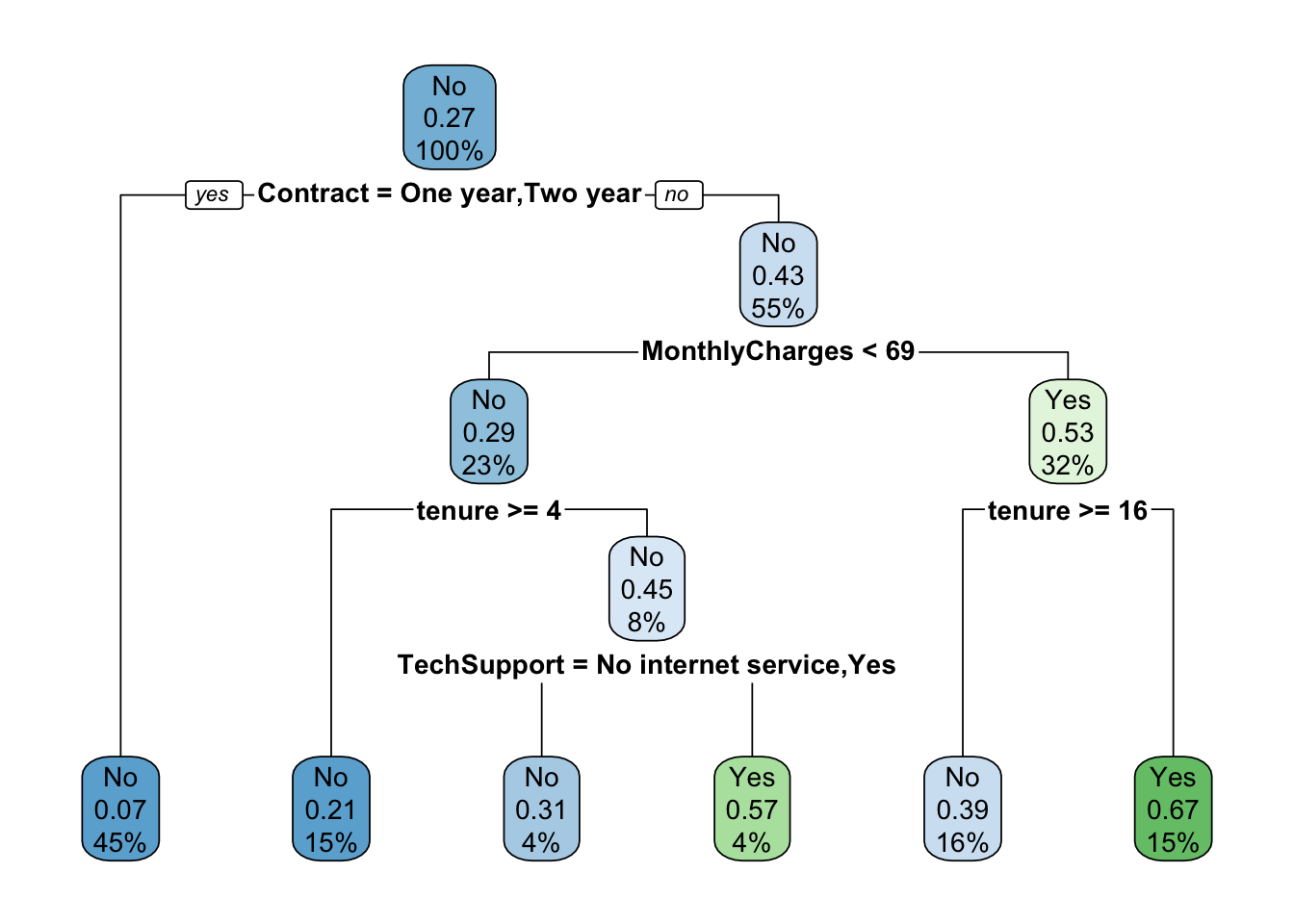``````train <- churn[indx,c("MonthlyCharges", "tenure", "TotalCharges", "Churn")]
test <- churn[-indx,c("MonthlyCharges", "tenure", "TotalCharges", "Churn")]

train <- na.omit(train)
test <- na.omit(test)
kn <- class::knn(train[,-4], test[,-4],train[,4] , k = 22, l = 4, prob = F, use.all = TRUE)
length(kn)``````
``##  1408``
``dim(test)``
``##  1408    4``
``````df <- table(Prob = kn, Obs = test[,"Churn"]) %>% data.frame()

ggplot(df, aes(x = Prob, y = Freq, fill = Obs)) +
geom_bar(stat = 'identity', position = "dodge") + theme_light()``````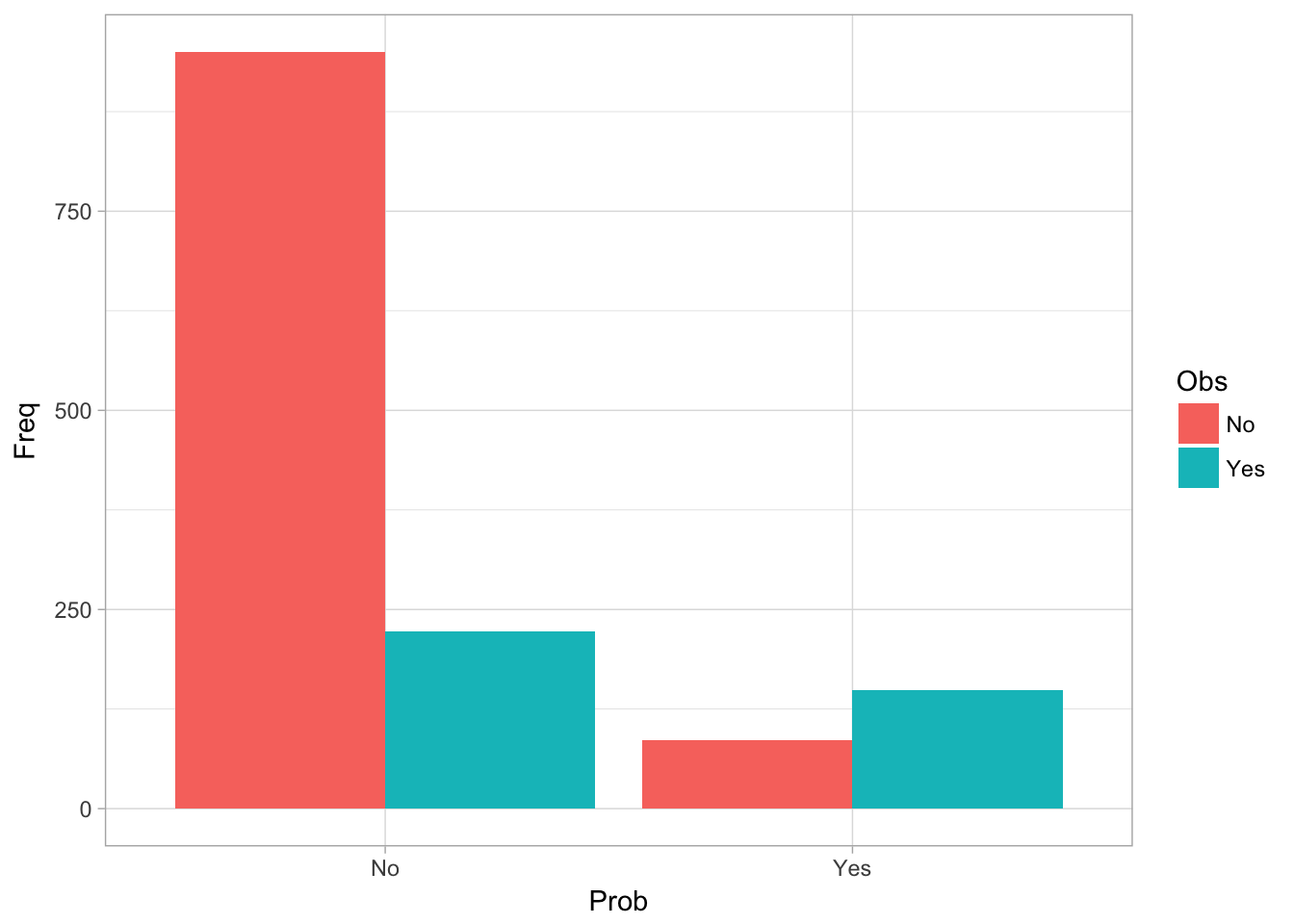``table(Prob = kn, Obs = test[,"Churn"])``
``````##      Obs
## Prob   No Yes
##   No  950 223
##   Yes  86 149``````
``table(Prob = kn, Obs = test[,"Churn"]) %>% prop.table()``
``````##      Obs
## Prob          No        Yes
##   No  0.67471591 0.15838068
##   Yes 0.06107955 0.10582386``````

This final model was able to achieve about 80% accuracy. KNN is very competitve with logistic regression and decision trees out of the box model.

## In God we trust. All others must bring data.

- W. Edwards Deming, Statistician